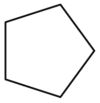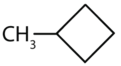# 12.9: Cycloalkanes

Skills to Develop

• To name cycloalkanes given their formulas and write formulas for these compounds given their names.

The hydrocarbons we have encountered so far have been composed of molecules with open-ended chains of carbon atoms. When a chain contains three or more carbon atoms, the atoms can join to form ring or cyclic structures. The simplest of these cyclic hydrocarbons has the formula C3H6. Each carbon atom has two hydrogen atoms attached (Figure $$\PageIndex{1}$$) and is called cyclopropane.Figure $$\PageIndex{1}$$: Ball-and-Spring Model of Cyclopropane. The springs are bent to join the carbon atoms.

## To Your Health: Cyclopropane as an Anesthetic

With its boiling point of −33°C, cyclopropane is a gas at room temperature. It is also a potent, quick-acting anesthetic with few undesirable side effects in the body. It is no longer used in surgery, however, because it forms explosive mixtures with air at nearly all concentrations.

The cycloalkanes—cyclic hydrocarbons with only single bonds—are named by adding the prefix cyclo- to the name of the open-chain compound having the same number of carbon atoms as there are in the ring. Thus the name for the cyclic compound C4H8 is cyclobutane. The carbon atoms in cyclic compounds can be represented by line-angle formulas that result in regular geometric figures. Keep in mind, however, that each corner of the geometric figure represents a carbon atom plus as many hydrogen atoms as needed to give each carbon atom four bonds.Some cyclic compounds have substituent groups attached. Example $$\PageIndex{1}$$ interprets the name of a cycloalkane with a single substituent group.

Example $$\PageIndex{1}$$

Draw the structure for each compound.

1. cyclopentane
2. methylcyclobutane

SOLUTION

1. The name cyclopentane indicates a cyclic (cyclo) alkane with five (pent-) carbon atoms. It can be represented as a pentagon.2. The name methylcyclobutane indicates a cyclic alkane with four (but-) carbon atoms in the cyclic part. It can be represented as a square with a CH3 group attached.Exercise $$\PageIndex{1}$$

Draw the structure for each compound.

1. cycloheptane
2. ethylcyclohexane

The properties of cyclic hydrocarbons are generally quite similar to those of the corresponding open-chain compounds. So cycloalkanes (with the exception of cyclopropane, which has a highly strained ring) act very much like noncyclic alkanes. Cyclic structures containing five or six carbon atoms, such as cyclopentane and cyclohexane, are particularly stable. We will see later that some carbohydrates (sugars) form five- or six-membered rings in solution.

The cyclopropane ring is strained because the C–C–C angles are 60°, and the preferred (tetrahedral) bond angle is 109.5°. (This strain is readily evident when you try to build a ball-and-stick model of cyclopropane; see Figure $$\PageIndex{1}$$.) Cyclopentane and cyclohexane rings have little strain because the C–C–C angles are near the preferred angles.

## Concept Review Exercises

1. What is the molecular formula of cyclooctane?

2. What is the IUPAC name for this compound?1. C8H16

2. ethylcyclopropane

## Key Takeaway

• Many organic compounds have cyclic structures.

## Exercises

1. Draw the structure for each compound.

1. ethylcyclobutane
2. propylcyclopropane
2. Draw the structure for each compound.

1. methylcyclohexane
2. butylcyclobutane
3. Cycloalkyl groups can be derived from cycloalkanes in the same way that alkyl groups are derived from alkanes. These groups are named as cyclopropyl, cyclobutyl, and so on. Name each cycloalkyl halide.

1.2.4. Halogenated cycloalkanes can be named by the IUPAC system. As with alkyl derivatives, monosubstituted derivatives need no number to indicate the position of the halogen. To name disubstituted derivatives, the carbon atoms are numbered starting at the position of one substituent (C1) and proceeding to the second substituted atom by the shortest route. Name each compound.

1.2.1.2.